# CHSE Odisha Class 12 Math Solutions Chapter 6 Probability Additional Exercise

Odisha State Board CHSE Odisha Class 12 Math Solutions Chapter 6 Probability Additional Exercise Textbook Exercise Questions and Answers.

## CHSE Odisha Class 12 Math Solutions Chapter 6 Probability Additional Exercise

(A) Multiple Choice Questions (Mcqs) With Answers

Question 1.
If $$\left|\begin{array}{ccc} 1+x & x & x^2 \\ x & 1+x & x^2 \\ x^2 & x & 1+x \end{array}\right|$$ = a + bx + cx2 + dx3 + ex4 + fx5 then write the value of a.
(a) 0
(b) 2
(c) 1
(d) 3
(c) 1

Question 2.
If every element of a third order determinant of value 8 is multiplied by 2, then write the value of the new determinant.
(a) 32
(b) 64
(c) 16
(d) 128
(b) 64Question 3.
If A is a 4 x 5 matrix and B is a matrix such that ATB and BAT both are defined, then write the order of B
(a) 4 x 5
(b) 1 x 5
(c) 5 x 4
(d) None of these
(a) 4 x 5

Question 4.
If $$\left[\begin{array}{lll} 3 & 5 & 3 \\ 2 & 4 & 2 \\ \lambda & 7 & 8 \end{array}\right]$$ is a singular matrix, write die value of 1.
(a) λ = 2
(b) λ = 1
(c) λ = 4
(d) λ = 8
(d) λ = 8

Question 5.
Determine the maximum value of $$\left|\begin{array}{rl} \cos x & \sin x \\ -\sin x & \cos x-1 \end{array}\right|$$
(a) 1
(b) 2
(c) 3
(d) 0
(b) 2

Question 6.
If $$\left[\begin{array}{cc} x & y \\ x & \frac{x}{2}+t \end{array}\right]$$ + $$\left[\begin{array}{cc} y & x+t \\ x+2 & \frac{x}{2} \end{array}\right]$$ = $$\left[\begin{array}{ll} 1 & 4 \\ 2 & 3 \end{array}\right]$$ then find x.
(a) x = 1
(b) x = 0
(c) x = 2
(d) x = -1
(b) x = 0

Question 7.
If $$\left[\begin{array}{cc} x & y \\ x & \frac{x}{2}+t \end{array}\right]$$ + $$\left[\begin{array}{cc} y & x+t \\ x+2 & \frac{x}{2} \end{array}\right]$$ = $$\left[\begin{array}{ll} 1 & 4 \\ 2 & 3 \end{array}\right]$$ then find y.
(a) y = 1
(b) y = 3
(c) y = 2
(d) y = 0
(a) y = 1

Question 8.
If $$\left[\begin{array}{cc} x & y \\ x & \frac{x}{2}+t \end{array}\right]$$ + $$\left[\begin{array}{cc} y & x+t \\ x+2 & \frac{x}{2} \end{array}\right]$$ = $$\left[\begin{array}{ll} 1 & 4 \\ 2 & 3 \end{array}\right]$$ then find t.
(a) t = 1
(b) t = 2
(c) t = 3
(d) t = 0
(c) t = 3Question 9.
Which matrix is a unit matrix?
(a) $$\left(\begin{array}{ll} 1 & 0 \\ 0 & 1 \end{array}\right)$$
(b) $$\left(\begin{array}{ll} 1 & 0 \\ 0 & 1 \end{array}\right)$$
(c) $$\left(\begin{array}{ll} 1 & 1 \\ 0 & 1 \end{array}\right)$$
(d) $$\left(\begin{array}{ll} 1 & 1 \\ 1 & 1 \end{array}\right)$$
(b) $$\left(\begin{array}{ll} 1 & 0 \\ 0 & 1 \end{array}\right)$$

Question 10.
If $$\left(\begin{array}{cc} \mathbf{x}_1 & \mathbf{x}_2 \\ \mathbf{y}_1 & \mathbf{y}_2 \end{array}\right)$$ – $$\left(\begin{array}{ll} 2 & 3 \\ 0 & 1 \end{array}\right)$$ = $$\left(\begin{array}{ll} 3 & 5 \\ 1 & 2 \end{array}\right)$$ then find x1, x2, y1, y2.
(a) x1 = 8, x2 = 5, y1 = 3, y2 = 1
(b) x1 = 1, x2 = 8, y1 = 5, y2 = 3
(c) x1 = 5, x2 = 8, y1 = 1, y2 = 3
(d) x1 = 3, x2 = 1, y1 = 8, y2 = 5
(c) x1 = 5, x2 = 8, y1 = 1, y2 = 3

Question 11.
If $$\left|\begin{array}{ll} 2 & 4 \\ k & 6 \end{array}\right|$$ = 0, what is the value of k?
(a) 3
(b) 4
(c) 2
(d) 6
(a) 3

Question 12.
If $$\left|\begin{array}{ll} \mathbf{a}_1 & \mathbf{b}_1 \\ \mathbf{c}_1 & \mathbf{d}_1 \end{array}\right|$$ = k $$\left|\begin{array}{ll} a_1 & c_1 \\ b_1 & d_1 \end{array}\right|$$ hen what is the value of k?
(a) 1
(b) 2
(c) 3
(d) 4
(d) 4

Question 13.
If A = $$\left(\begin{array}{lll} 1 & 0 & 2 \\ 5 & 1 & x \\ 1 & 1 & 1 \end{array}\right)$$ is a singular matrix then what is the value of x?
(a) 6
(b) 7
(c) 8
(d) 9
(d) 9

Question 14.
Evaluate $$\left|\begin{array}{ccc} -6 & 0 & 0 \\ 3 & -5 & 7 \\ 2 & 8 & 11 \end{array}\right|$$
(a) 66
(b) 666
(c) 6666
(d) 6
(b) 666

Question 15.
Evaluate $$\left|\begin{array}{lll} 1 & 1 & b+c \\ 1 & b & c+a \\ 1 & c & a+b \end{array}\right|$$
(a) 0
(b) 1
(c) 11
(d) 2
(a) 0Question 16.
Evaluate $$\left|\begin{array}{ccc} 1^2 & 2^2 & 3^2 \\ 2^2 & 3^2 & 4^2 \\ 3^2 & 4^2 & 5^2 \end{array}\right|$$
(a) 54
(b) 58
(c) -54
(d) 60
(c) -54

Question 17.
If A and B are square matrices of order 3, such that |A| = -1, |B| = 3 then |3 AB| = –
(a) 1
(b) 11
(c) 9
(d) 81
(d) 81

Question 18.
For what k
x + 2y – 3z = 2
(k + 3)z = 3
(2k + 1)y + z = 2 is inconsistent?
(a) -3
(b) -6
(c) 3
(d) 6
(a) -3

Question 19.
The sum of two nonintegral roots of $$\left|\begin{array}{lll} x & 2 & 5 \\ 3 & x & 3 \\ 5 & 4 & x \end{array}\right|$$ = 0 is ______.
(a) 5
(b) -5
(c) 3
(d) 15
(b) -5

Question 20.
The value of $$\left|\begin{array}{ccc} 1 & 2 & 3 \\ 3 & 5 & 2 \\ 8 & 14 & 20 \end{array}\right|$$ is ______.
(a) 1
(b) 2
(c) 0
(d) 3
(c) 0

Question 21.
If [x 1] $$\left[\begin{array}{cc} 1 & 0 \\ -2 & 0 \end{array}\right]$$ = 0, then x equals:
(a) 0
(b) -2
(c) -1
(d) 2
(d) 2

Question 22.
The number of all possible matrices of order 3 × 3 with each entry 0 or 1 is:
(a) 27
(b) 18
(c) 81
(d) 512
(d) 512

Question 23.
If A = $$\left[\begin{array}{cc} \cos \alpha & -\sin \alpha \\ \sin \alpha & \cos \alpha \end{array}\right]$$ , and A + A’ = I, then the value of α is
(a) $$\frac{\pi}{6}$$
(b) $$\frac{\pi}{3}$$
(c) π
(d) $$\frac{3 \pi}{2}$$
(b) $$\frac{\pi}{3}$$

Question 24.
Matrix A and B will be inverse of each other only if
(a) AB = BA
(b) AB = BA = 0
(c) AB = 0, BA = I
(d) AB = BA = I
(d) AB = BA = I

Question 25.
The matrix P = $$\left[\begin{array}{lll} 0 & 0 & 4 \\ 0 & 4 & 0 \\ 4 & 0 & 0 \end{array}\right]$$ is a
(a) square matrix
(b) diagonal matrix
(c) unit matrix
(d) None of these
(a) square matrixQuestion 26.
If A and B are symmetric matrices of same order, then AB – BA is a
(a) Skew-symmetric matrix
(b) Symmetric matrix
(c) Zero matrix
(d) Identity
(a) Skew-symmetric matrix

Question 27.
If A is a square matrix of order 3, such that A(adj A) = 10I, then |adj A| is equal to
(a) 1
(b) 10
(c) 100
(d) 1000
(c) 100

Question 28.
Let A be a square matrix of order 2 × 2, then |KA| is equal to
(a) K|A|
(b) K2|A|
(c) K3|A|
(d) 2K|A|
(b) K2|A|

Question 29.
If A and B are invertible matrices then which of the following is not correct
(a) Adj A = |A|. A-1
(b) det (A-1) = (det A)-1
(c) (AB)-1 = B-1A-1
(d) (A + B)-1 = A-1 + B-1
(d) (A + B)-1 = A-1 + B-1

Question 30.
If A is a skew-symmetric matrix of order 3, then the value of |A| is
(a) 3
(b) 0
(c) 9
(d) 27
(b) 0

Question 31.
If A is a square matrix of order 3, such that A(adjA) = 10I, then ladj Al is equal to
(a) 1
(b) 10
(c) 100
(d) 1000
(c) 100

Question 32.
Let A be a non-angular square matrix of order 3 x 3, then |A. adj Al is equal to
(a) |A|3
(b) |A|2
(c) |A|
(d) 3|A|
(a) |A|3

Question 33.
Let A be a square matrix of order 3 × 3 and k a scalar, then |kA| is equal to
(a) k|A|
(b) |k||A|
(c) k3|A|
(d) none of these
(c) k3|A|

Question 34.
If a, b, c are all distinct, and $$\left|\begin{array}{lll} a & a^2 & 1+a^3 \\ b & b^2 & 1+b^3 \\ c & c^2 & 1+c^3 \end{array}\right|$$ = 0 then the value of abc is
(a) 0
(b) -1
(c) 3
(d) -3
(b) -1

Question 35.
If a, b, c are in AP, then the value of $$\left|\begin{array}{lll} x+1 & x+2 & x+a \\ x+2 & x+3 & x+b \\ x+3 & x+4 & x+c \end{array}\right|$$ is:
(a) 4
(b) -3
(c) 0
(d) abc
(c) 0

Question 36.
If A is a skew-symmetric matrix of order 3, then the value of |A| is
(a) 3
(b) 0
(c) 9
(d) 27
(b) 0Question 37.
A bag contains 3 white, 4 black and 2 red balls. If 2 balls are choosen at random (without replacement), then the probability that both the balls are white is:
(a) $$\frac{1}{18}$$
(b) $$\frac{2}{9}$$
(c) $$\frac{1}{12}$$
(d) $$\frac{1}{24}$$
(c) $$\frac{1}{12}$$

Question 38.
Three diece are thrown simultaneously. The probability of obtaining a total score of 5 is:
(a) $$\frac{5}{216}$$
(b) $$\frac{1}{6}$$
(c) $$\frac{1}{36}$$
(d) $$\frac{1}{49}$$
(c) $$\frac{1}{36}$$

Question 39.
An urn contains 6 balls of which two are red and four are black. Two balls are drawn at random. Probability that they are of the different colour is:
(a) $$\frac{2}{5}$$
(b) $$\frac{1}{15}$$
(c) $$\frac{8}{15}$$
(d) $$\frac{4}{15}$$
(d) $$\frac{4}{15}$$

Question 40.
The probability of obtaining an even prime number on each die when a pair of dice is rolled is:
(a) 0
(b) $$\frac{1}{3}$$
(c) $$\frac{1}{12}$$
(d) $$\frac{1}{36}$$
(d) $$\frac{1}{36}$$

Question 41.
Two events A and B are said to be independent if:
(a) A and B are mutually exclusive
(b) P (A’B’) = [1 – P(A)][1 – P(B)]
(c) P(A) = P(B)
(d) P(A) + P(B) = 1
(b) P (A’B’) = [1 – P(A)][1 – P(B)]

Question 42.
A die is. thrown once, then the probability of getting number greater than 3 is:
(a) $$\frac{1}{2}$$
(b) $$\frac{2}{3}$$
(c) 6
(d) 0
(a) $$\frac{1}{2}$$

Question 43.
If P(A) = $$\frac{6}{11}$$, P(B) = $$\frac{5}{11}$$ and P(A ∪ B) = $$\frac{7}{11}$$, then P(A/B) is:
(a) $$\frac{2}{5}$$
(b) $$\frac{3}{5}$$
(c) $$\frac{4}{5}$$
(d) 1
(c) $$\frac{4}{5}$$

Question 44.
Let the target be hit A and B: the target be hit by B and C: the target be hit by A and C. Then the probability that A, B and C all will hit, is:
(a) $$\frac{4}{5}$$
(b) $$\frac{3}{5}$$
(c) $$\frac{2}{5}$$
(d) $$\frac{1}{5}$$
(c) $$\frac{2}{5}$$

Question 45.
What is the probability that ‘none of them will hit the target’?
(a) $$\frac{1}{30}$$
(b) $$\frac{1}{60}$$
(c) $$\frac{1}{15}$$
(d) $$\frac{2}{15}$$
(b) $$\frac{1}{60}$$(B) Very Short Type Questions With Answers

Question 1.
If $$\left|\begin{array}{ccc} 1+\mathbf{x} & \mathbf{x} & \mathbf{x}^2 \\ \mathbf{x} & 1+\mathbf{x} & \mathbf{x}^2 \\ \mathbf{x}^2 & \mathbf{x} & 1+\mathbf{x} \end{array}\right|$$ = a + bx + cx2 + dx3 + ex4 + fx5 then write the value of a.
Solution:
$$\left|\begin{array}{ccc} 1+\mathbf{x} & \mathbf{x} & \mathbf{x}^2 \\ \mathbf{x} & 1+\mathbf{x} & \mathbf{x}^2 \\ \mathbf{x}^2 & \mathbf{x} & 1+\mathbf{x} \end{array}\right|$$
= a + bx + cx2 + dx3 + ex4 + fx5
which is an identity
Putting x = 0 we get
a = $$\left|\begin{array}{lll} 1 & 0 & 0 \\ 0 & 1 & 0 \\ 0 & 0 & 1 \end{array}\right|$$ = 1

Question 2.
If every element of a third order determinant of value 8 is multiplied by 2, then write the value of the new determinant.
Solution:
According to the question
|A| = 8
Now |KA| = Kn|A|
⇒ |2A| = 23|A| = 8 × 8 = 64
Value of the new determinant is 64.

Question 3.
If I is an identity matrix of order n, then k being a natural number, write the matrix Ikn.
Solution:
If I is an identity matrix of order n, then Ikn = In

Question 4.
If A is a 4 × 5 matrix and B is a matrix such that ATB and BAT both are defined, then write the order of B.
Solution:
Order of A = 4 × 5
Order of AT = 5 × 4
Let order of B = m × n
ATB is well defined ⇒ m = 4
BAT is well defined ⇒ n = 5
Order of B = 4 × 5

Question 5.
Write the matrix which when added to the matrix $$\left[\begin{array}{cc} 2 & -3 \\ -4 & 7 \end{array}\right]$$ gives the matrix $$\left[\begin{array}{ll} 4 & 1 \\ 3 & 2 \end{array}\right]$$
Solution:
Let the required matrix is A.
$$\left(\begin{array}{cc} 2 & -3 \\ -4 & 7 \end{array}\right)$$ + A = $$\left(\begin{array}{ll} 4 & 1 \\ 3 & 2 \end{array}\right)$$
A = $$\left(\begin{array}{ll} 4 & 1 \\ 3 & 2 \end{array}\right)$$ – $$\left(\begin{array}{cc} 2 & -3 \\ -4 & 7 \end{array}\right)$$ = $$\left(\begin{array}{cc} 2 & 4 \\ 7 & -5 \end{array}\right)$$

Question 6.
Determine the maximum value of $$\left|\begin{array}{rl} \cos x & \sin x \\ -\sin x & \cos x-1 \end{array}\right|$$
Solution:
Let f(x) = $$\left|\begin{array}{rl} \cos x & \sin x \\ -\sin x & \cos x-1 \end{array}\right|$$
= cos2x – cos x + sin2x = 1 – cos x
As – 1 < cos x ≤ 1
⇒ 1 >- cos x ≥ – 1
⇒ 2 > 1 – cos x ≥ 0
The maximum value of f(x) = 2.Question 7.
Write the value of k if:
$$\left|\begin{array}{lll} \mathbf{a a _ { 1 }} & \mathbf{a a}_2 & \mathbf{a} \mathbf{a}_3 \\ \mathbf{a b _ { 1 }} & \mathbf{a b}_2 & \mathbf{a b} \\ \mathbf{a c _ { 2 }} & \mathbf{a c}_2 & \mathbf{a c _ { 3 }} \end{array}\right|$$ = k$$\left|\begin{array}{lll} \mathbf{a}_1 & \mathbf{b}_1 & \mathbf{c}_1 \\ \mathbf{a}_2 & \mathbf{b}_2 & \mathbf{c}_2 \\ \mathbf{a}_3 & \mathbf{b}_3 & \mathbf{c}_3 \end{array}\right|$$
Solution:
$$\left|\begin{array}{lll} \mathbf{a a _ { 1 }} & \mathbf{a a}_2 & \mathbf{a} \mathbf{a}_3 \\ \mathbf{a b _ { 1 }} & \mathbf{a b}_2 & \mathbf{a b} \\ \mathbf{a c _ { 2 }} & \mathbf{a c}_2 & \mathbf{a c _ { 3 }} \end{array}\right|$$ = k$$\left|\begin{array}{lll} \mathbf{a}_1 & \mathbf{b}_1 & \mathbf{c}_1 \\ \mathbf{a}_2 & \mathbf{b}_2 & \mathbf{c}_2 \\ \mathbf{a}_3 & \mathbf{b}_3 & \mathbf{c}_3 \end{array}\right|$$
k = a3.

Question 8.
If A is a 3 × 3 matrix and |A| = 3, then write the matrix represented by A × adj A.
Solution:
|A| = 3 ⇒ A × Adj A = $$\left(\begin{array}{lll} 3 & 0 & 0 \\ 0 & 3 & 0 \\ 0 & 0 & 3 \end{array}\right)$$

Question 9.
If ω is a complex cube root of 1, then for what value of λ the determinant
$$\left|\begin{array}{ccc} 1 & \omega & \omega^2 \\ \omega & \lambda & 1 \\ \omega^2 & 1 & \omega \end{array}\right|$$ = 0?
Solution: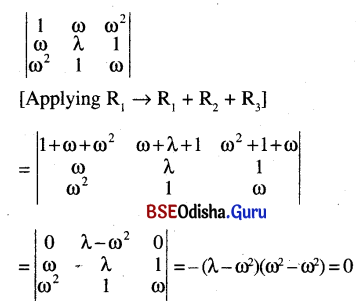⇒ for any value of ‘A,’ the given determinant is ‘0’

Question 10.
If [1 2 3] A = , then what is the der of the matrix A?
Solution:
If [1 2 3] A = 
A is a 3 × 1 matrix

Question 11.
What is A + B if A = $$\left(\begin{array}{cc} 1 & 2 \\ 3 & -1 \end{array}\right)$$, B = $$\left(\begin{array}{cc} 0 & -1 \\ -2 & 1 \end{array}\right)$$?
Solution:
For A = $$\left(\begin{array}{cc} 1 & 2 \\ 3 & -1 \end{array}\right)$$, B = $$\left(\begin{array}{cc} 0 & -1 \\ -2 & 1 \end{array}\right)$$
A + B = $$\left(\begin{array}{ll} 1 & 1 \\ 1 & 0 \end{array}\right)$$

Question 12.
Give an example of a unit matrix.
Solution:
$$\left(\begin{array}{ll} 1 & 0 \\ 0 & 1 \end{array}\right)$$ is a unit matrix of 2nd order.Question 13.
Construct a 2 × 3 matrix having elements defined by aij = i – j.
Solution:
aij = i – j
a11 =0, a12 = 1 – 2 = – 1, a13 = 1 – 3 =- 2
a21 = 2 – 1 = 1, a22 = 2 – 2 = 0, a23 = 2 – 3 = -1
∴ The required matrix is $$\left(\begin{array}{ccc} 0 & -1 & -2 \\ 0 & 0 & -1 \end{array}\right)$$.

Question 14.
Find x, y if A = A’ where A = $$\left(\begin{array}{ll} 5 & \mathbf{x} \\ \mathbf{y} & 0 \end{array}\right)$$
Solution:
A = A’
⇒ $$\left(\begin{array}{ll} 5 & \mathbf{x} \\ \mathbf{y} & 0 \end{array}\right)$$ = $$\left(\begin{array}{ll} 5 & \mathbf{y} \\ \mathbf{x} & 0 \end{array}\right)$$ ⇒ x = y
∴ x and y are any real number where x = y

Question 15.
Cana matrix be constructed by taking 29 elements?
Solution:
Only two matrices can be formed by taking 29 elements. They are of order 1 × 29 and 29 × 1.

Question 16.
If $$\left|\begin{array}{ll} 2 & 4 \\ k & 6 \end{array}\right|$$ = 0 , what is the value of k?
Solution:
$$\left|\begin{array}{ll} 2 & 4 \\ k & 6 \end{array}\right|$$ = 0 ⇒ 12 – 4k = 0 ⇒ k = 3

Question 17.
If $$\left|\begin{array}{ll} \mathbf{a}_1 & \mathbf{b}_1 \\ \mathbf{c}_{\mathbf{1}} & \mathbf{d}_1 \end{array}\right|$$ = k = $$\left|\begin{array}{ll} \mathbf{a}_1 & \mathbf{c}_1 \\ \mathbf{b}_1 & \mathbf{d}_1 \end{array}\right|$$ then what is the value of k?
Solution:
k = 1

Question 18.
If A and B are square matrices of order 3, such that |A| = -1, |B| = 3 then |3 AB| = ______.
Solution:
|3 AB| = 27 |A| |B| = 81

Question 19.
Solve: $$\left|\begin{array}{ccc} 2 & 2 & x \\ -1 & x & 4 \\ 1 & 1 & 1 \end{array}\right|$$ = 0
Solution:
$$\left|\begin{array}{ccc} 2 & 2 & x \\ -1 & x & 4 \\ 1 & 1 & 1 \end{array}\right|$$ = 0 => $$\left|\begin{array}{ccc} 0 & 2 & x \\ -1-x & x & 4 \\ 0 & 1 & 1 \end{array}\right|$$ = 0
⇒ – (- 1 – x) (2 – x) = 0 ⇒ x = -1, x = 2.(C) Short Type Questions With Answers

Question 1.
If A = $$\left[\begin{array}{ccc} 1 & 2 & 3 \\ 3 & -2 & 1 \\ 4 & 2 & 1 \end{array}\right]$$ then show that A3 – 23A – 40I = 0
Solution: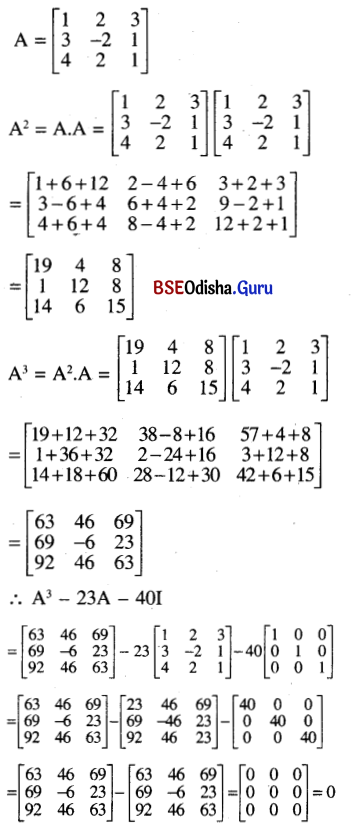Question 2.
Solve: $$\left|\begin{array}{ccc} \mathbf{x + 1} & \omega & \omega \\ \omega & x+\omega^2 & 1 \\ \omega^2 & 1 & x+\omega \end{array}\right|$$ = 0
Solution: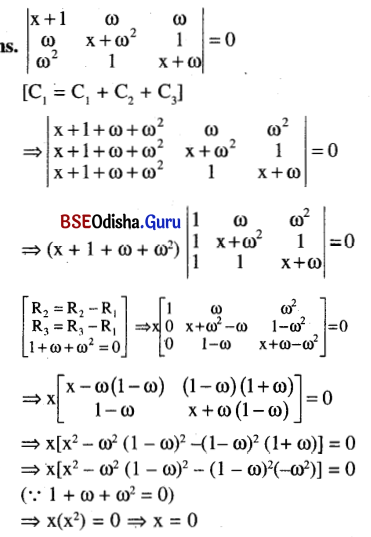Question 3.
If A = $$\left[\begin{array}{ccc} 1 & 2 & 0 \\ 0 & 1 & 3 \\ -2 & 5 & 3 \end{array}\right]$$, then verify that A + A’ is symmetric and A – A’ is skew symmetric.
Solution: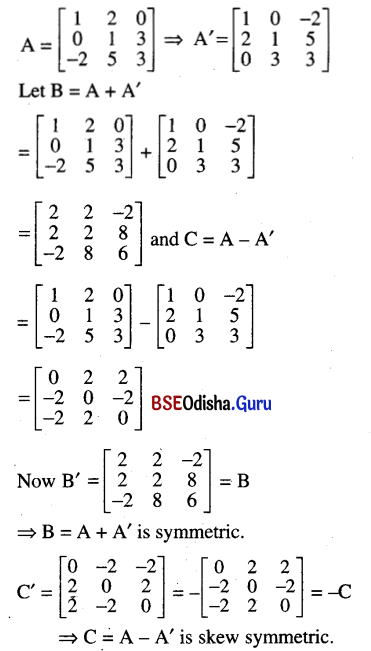Question 4.
If A, B, C are matrices of order 2 × 2 each and 2A + B + C = $$\left[\begin{array}{ll} 1 & 2 \\ 3 & 0 \end{array}\right]$$, A + B + C = $$\left[\begin{array}{ll} 0 & 1 \\ 2 & 1 \end{array}\right]$$ and A + B – C = $$\left[\begin{array}{ll} 1 & 2 \\ 1 & 0 \end{array}\right]$$, then find A, B and C.
Solution: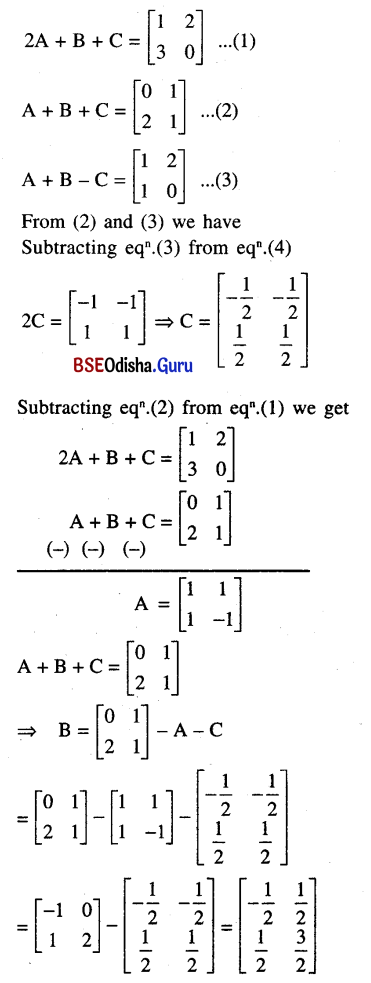Question 5.
Find the inverse of the following matrix: $$\left[\begin{array}{lll} 1 & 1 & 2 \\ 0 & 1 & 2 \\ 1 & 2 & 1 \end{array}\right]$$
Solution:
Let A = $$\left[\begin{array}{lll} 1 & 1 & 2 \\ 0 & 1 & 2 \\ 1 & 2 & 1 \end{array}\right]$$
Method – I
Let us find A-1 by using elementary row transformation.
Let A = IA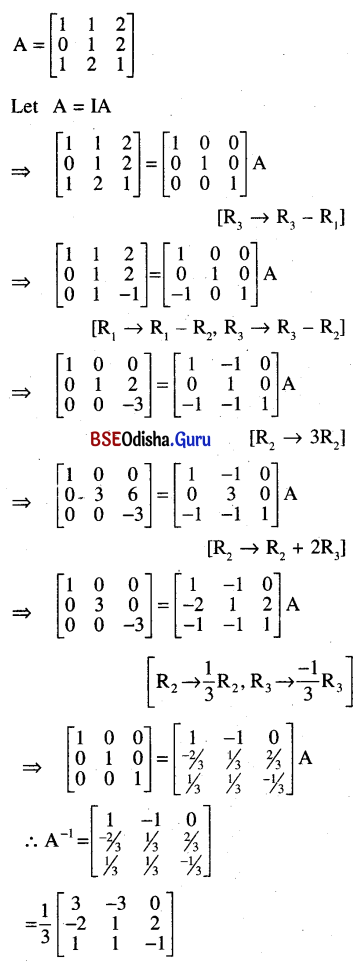Method – II
|A| = 1(1 – 4) – 1(0- 2) + 2(0- 1)
= 1(-3) – 1(-2) + 2(-1)
= -3 ≠ 0
∴ A-1 exists.
A11 = -3, A12 = 2, A13 = -1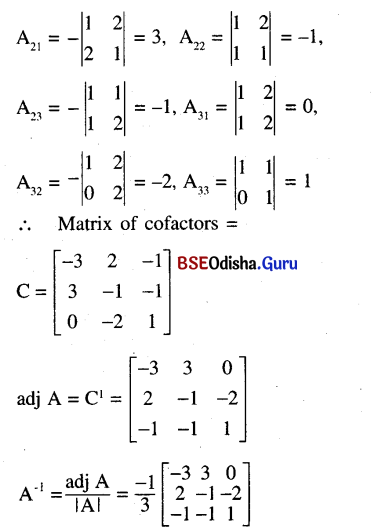Question 6.
Show that $$\left|\begin{array}{ccc} a-b-c & 2 a & 2 a \\ 2 b & b-c-a & 2 b \\ 2 c & 2 c & c-a-b \end{array}\right|$$ = (a+b +c)3
Solution: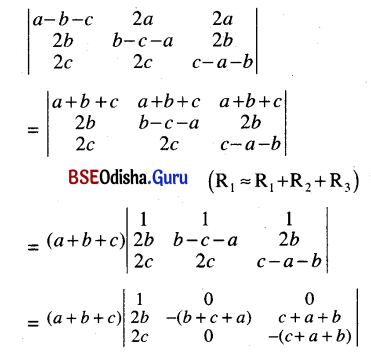= (a + b + c)3 (1 – 0) = (a + b + c)3

Question 7.
Find the inverse of the following matrix: $$\left[\begin{array}{lll} 0 & 0 & 2 \\ 0 & 2 & 0 \\ 2 & 0 & 0 \end{array}\right]$$
Solution:
A = $$\left[\begin{array}{lll} 0 & 0 & 2 \\ 0 & 2 & 0 \\ 2 & 0 & 0 \end{array}\right]$$
|A| = 2(- 4) = – 8 ≠ 0
∴ A-1 exists.
A11 = 0, A12 = 0, A13 = – 4
A21 = 0, A22 = – 4, A23 = 0
A31 = 0,A32 = 0, A33 = – 4
∴ The matrix of cofactors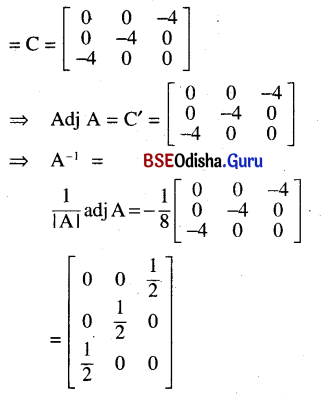Question 8.
If the matrix A is such that $$\left[\begin{array}{cc} 1 & -1 \\ 2 & 3 \end{array}\right]$$A = $$\left[\begin{array}{cc} -4 & 1 \\ 7 & 7 \end{array}\right]$$, find A.
Solution: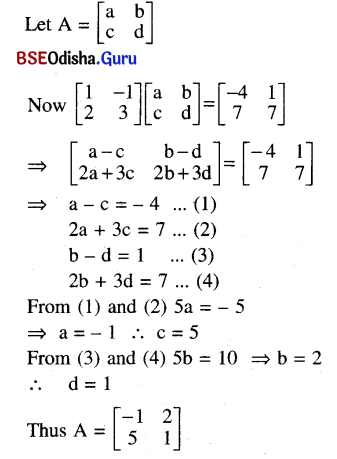Question 9.
Show that (a + 1) is a factor of $$\left|\begin{array}{ccc} a+1 & 2 & 3 \\ 1 & a+1 & 3 \\ 3 & -6 & a+1 \end{array}\right|$$.
Solution: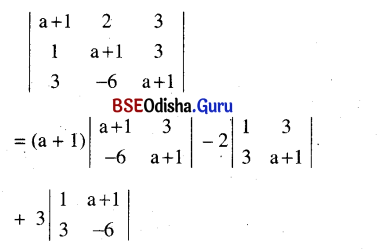= (a + 1) (a2 + 2a + 1 + 18) – 2(a + 1 – 9) + 3(-6 – 3a – 3)
= (a + 1)(a2 + 2a + 19) – 2a + 16 – 27 – 9a
= (a + 1) (a2 + 2a + 19) – 11a – 11
= (a + 1) (a2 + 2a + 19) – 11(a + 1)
= (a + 1) (a2 + 2a + 8)
⇒ (a + 1) is a factor of the given determinant.Question 11.
If A = $$\left[\begin{array}{ll} \alpha & 0 \\ 1 & 1 \end{array}\right]$$ and B = $$\left[\begin{array}{ll} 1 & 0 \\ 5 & 1 \end{array}\right]$$ show that for no values of α, A2 = B.
Solution: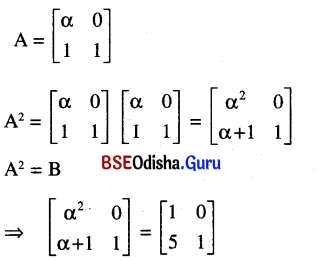⇒ α2 = 1 and α + 1 = 5
⇒ α = ± 1 and α = 4
Which is not possible.
There is no α for which A2 = B

Question 12.
If A = $$\left[\begin{array}{ll} 3 & -4 \\ 1 & -1 \end{array}\right]$$, then show that Ak = $$\left[\begin{array}{cc} 1+2 \mathrm{k} & -4 \mathrm{k} \\ \mathrm{k} & 1-2 \mathrm{k} \end{array}\right]$$, k ∈ N.
Solution: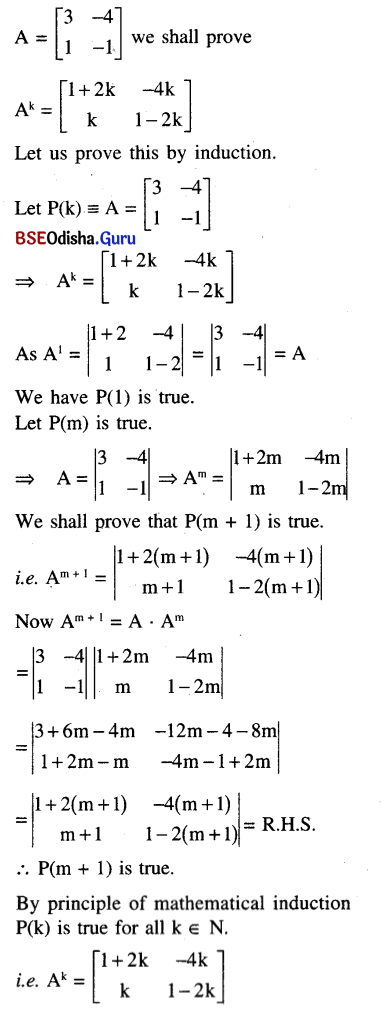Question 13.
If A = $$\left[\begin{array}{ccc} 1 & -2 & 2 \\ 3 & 1 & -1 \end{array}\right]$$, B = $$\left[\begin{array}{cc} 2 & 4 \\ 1 & 2 \\ 3 & -1 \end{array}\right]$$, verify that (AB)T = BTAT.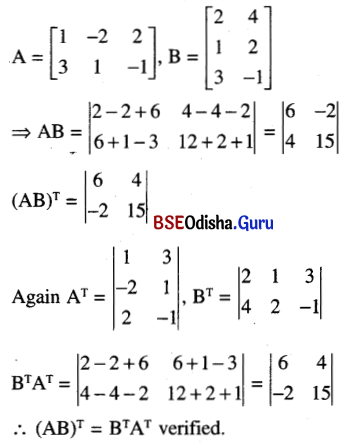Question 14.
Show that for each real value of λ the system of equations
(λ + 3) + λy = 0
x + (2λ + 5)y = 0 has a unique solution.
Solution:
Given system of equations is a
homogeneous system of linear
equations.
Now
Δ = $$\left|\begin{array}{cc} \lambda+3 & \lambda \\ 1 & 2 \lambda+5 \end{array}\right|$$
= (λ + 3)(2λ + 5) – λ
= 2λ2 + 11λ + 15 – λ
= 2λ2 + 10λ + 15
As for 2λ2 + 10A + 15, D = 100 – 120 < 0
the polynomial 2λ2 + 10λ + 15 has no roots i.e. Δ ≠ 0.
Thus the system has a unique trivial solution for every real value of λ.

Question 15.
If A and B are square matrices of same order then show by means of an example that AB ≠ BA in general.
Solution: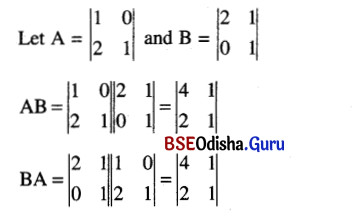∴ AB ≠ BA we have AB ≠ BA in general.

Question 16.
If A = $$\left|\begin{array}{cc} 0 & -\tan \frac{\theta}{2} \\ \tan \frac{\theta}{2} & 0 \end{array}\right|$$, then prove that det{(I + A)(I – A)-1} = 1
Solution: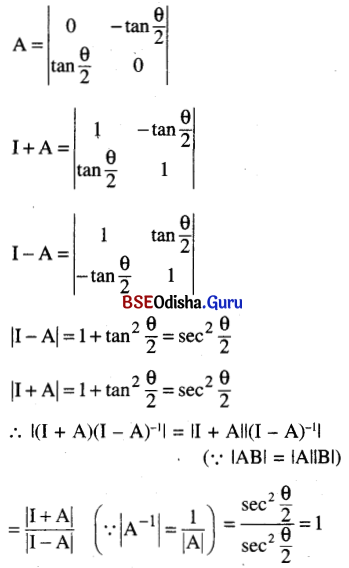Question 17.
Solve for x: $$\left|\begin{array}{ccc} 15-2 x & 11 & 10 \\ 11-3 x & 17 & 16 \\ 7-x & 14 & 13 \end{array}\right|$$ = 0
Solution: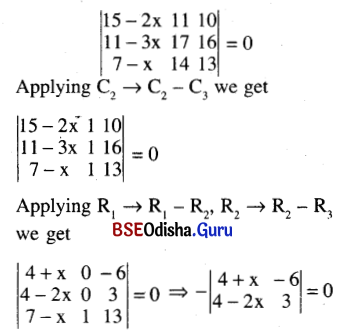Question 18.
If A = $$\left[\begin{array}{ccc} -1 & 3 & 5 \\ 1 & -3 & -5 \\ -1 & 3 & 5 \end{array}\right]$$ find A3 – A2.
Solution: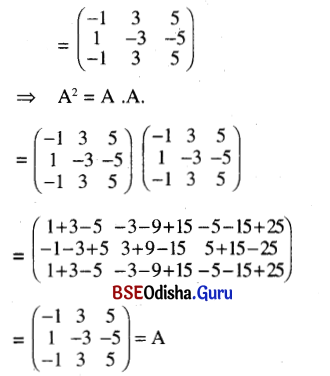A2 = A ⇒ A2A = A2
⇒ A3 – A2 = 0

Question 19.
Prove that: A = $$\left|\begin{array}{ccc} 2 & 3 & 4 \\ 1 & -2 & -3 \\ 3 & 1 & -8 \end{array}\right|$$ ⇒ A2 – 5A + 71 = 0.
Solution: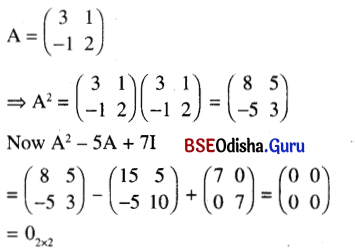Question 20.
Test whether the following system of equations have non-zero solution.
Write the solution set:
2x + 3y + 4z = 0,
x – 2y – 3z = 0,
3x + y – 8z = 0.
Solution:
Given equations are
2x + 3y + 4z = 0
x – 2y – 3z = 0
3x + y – 8z = 0
Now $$\left|\begin{array}{ccc} 2 & 3 & 4 \\ 1 & -2 & -3 \\ 3 & 1 & -8 \end{array}\right|$$
= 2(19) – 3(1) + 4(7) 0
∴ The system has no non-zero solution.
The solution set is x = 0; y = 0, z = 0.